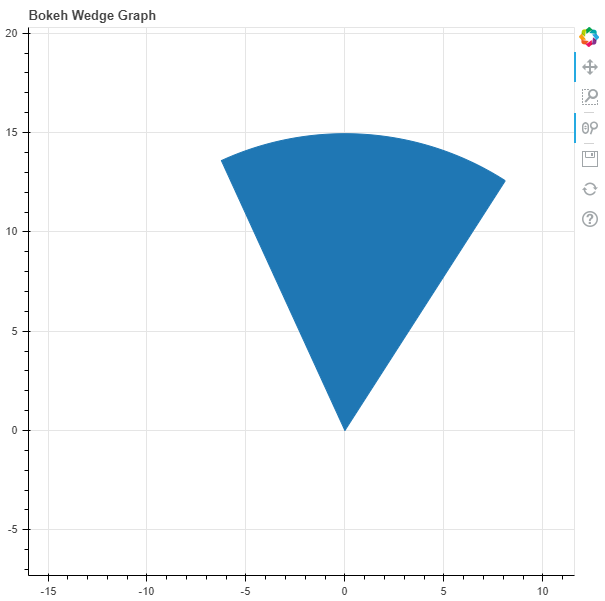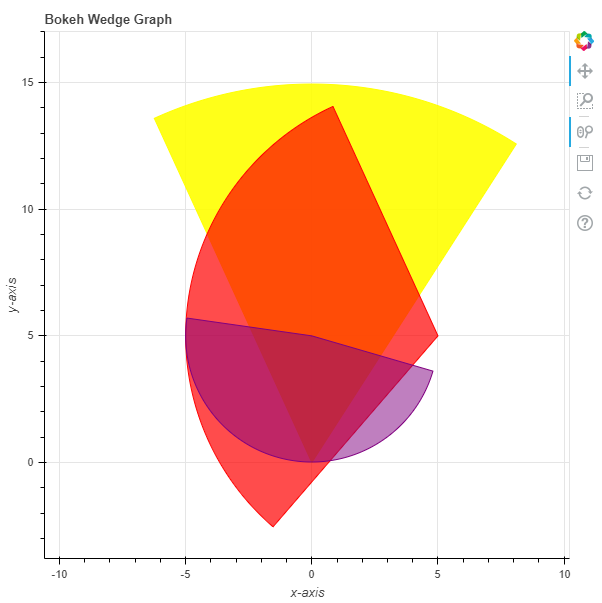Open in App
Not now

# Python Bokeh – Plotting Wedges on a Graph

• Last Updated : 10 Jul, 2020

Bokeh is a Python interactive data visualization. It renders its plots using HTML and JavaScript. It targets modern web browsers for presentation providing elegant, concise construction of novel graphics with high-performance interactivity.

Bokeh can be used to plot wedges on a graph. Plotting wedges on a graph can be done using the `wedge()` method of the `plotting` module.

## plotting.figure.wedge()

Syntax : wedge(parameters)

Parameters :

• x : x-coordinates of the wedge markers
• y : y-coordinates of the wedge markers
• start_angle : angle to start the wedge markers
• start_angle_units : unit of the angle to start the wedge markers, default is radian
• end_angle : angle to end the wedge markers
• end_angle_units : unit of the angle to end the wedge markers
• direction : direction of strokes, default is anticlock

Returns : an object of class `GlyphRenderer`

Example 1 : In this example we will be using the default values for plotting the graph.

 `# importing the modules ` `from` `bokeh.plotting ``import` `figure, output_file, show ` `        `  `# file to save the model ` `output_file(``"gfg.html"``) ` `        `  `# instantiating the figure object ` `graph ``=` `figure(title ``=` `"Bokeh Wedge Graph"``) ` `      `  `# the points to be plotted ` `x ``=` `0` `y ``=` `0` ` `  `# radius of the wedge ` `radius ``=` `15` ` `  `# start angle of the wedge ` `start_angle ``=` `1` ` `  `# end angle of the wedge ` `end_angle ``=` `2` ` `  `# plotting the graph ` `graph.wedge(x, y, ` `            ``radius ``=` `radius, ` `            ``start_angle ``=` `start_angle, ` `            ``end_angle ``=` `end_angle) ` `      `  `# displaying the model ` `show(graph) `

Output :Example 2 : In this example we will be plotting multiple wedges with various other parameters.

 `# importing the modules ` `from` `bokeh.plotting ``import` `figure, output_file, show ` `        `  `# file to save the model ` `output_file(``"gfg.html"``) ` `        `  `# instantiating the figure object ` `graph ``=` `figure(title ``=` `"Bokeh Wedge Graph"``) ` ` `  `# name of the x-axis  ` `graph.xaxis.axis_label ``=` `"x-axis"` `       `  `# name of the y-axis  ` `graph.yaxis.axis_label ``=` `"y-axis"` ` `  `# the points to be plotted ` `x ``=` `[``0``, ``5``, ``0``] ` `y ``=` `[``0``, ``5``, ``5``] ` ` `  `# radius of the wedges ` `radius ``=` `[``15``, ``10``, ``5``] ` ` `  `# start angle of the wedges ` `start_angle ``=` `[``1``, ``2``, ``3``] ` ` `  `# end angle of the wedges ` `end_angle ``=` `[``2``, ``4``, ``6``] ` ` `  `# color value of the wedges ` `color ``=` `[``"yellow"``, ``"red"``, ``"purple"``] ` `  `  `# fill alpha value of the wedges ` `fill_alpha ``=` `[``0.9``, ``0.7``, ``0.5``] ` ` `  `# plotting the graph ` `graph.wedge(x, y, ` `            ``radius ``=` `radius, ` `            ``start_angle ``=` `start_angle, ` `            ``end_angle ``=` `end_angle, ` `            ``color ``=` `color, ` `            ``fill_alpha ``=` `fill_alpha) ` `      `  `# displaying the model ` `show(graph) `

Output :My Personal Notes arrow_drop_up
Related Articles